# Sum of odd numbers

Find the sum of all odd integers from 13 to 781.

s =  152845

### Step-by-step explanation: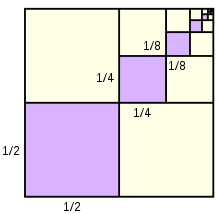Did you find an error or inaccuracy? Feel free to write us. Thank you!## Related math problems and questions:

• Find the sumFind the sum of all natural numbers from 1 and 100, which are divisible by 2 or 5
• Gauss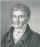Help little C.F. Gauss sum all the integers from 1 to 400.
• Odd numbersThe sum of four consecutive odd numbers is 1048. Find those numbers ...
• Sum of inner angles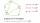Prove that the sum of all inner angles of any convex n-angle equals (n-2) . 180 degrees.
• The sum 2The sum of five consecutive even integers is 150. Find the largest of the five integers. A.28 B.30 C.34 D.54 Show your solution and explain your answer.
• Three members GPThe sum of three numbers in GP (geometric progression) is 21 and the sum of their squares is 189. Find the numbers.
• Sum of the seventeen numbersThe sum of the 17 different natural numbers is 154. Determine the sum of the two largest ones.
• Sum of sevenThe sum of seven consecutive odd natural numbers is 119. Determine the smallest of them.
• Sum 1-6Find the sum of the geometric progression 3, 15, 75,… to six terms.
• Finite arithmetic sequenceHow many numbers should be inserted between the numbers 1 and 25 so that all numbers create a finite arithmetic sequence and that the sum of all members of this group is 117?
• Three integersThree consecutive integers add up to 51. what are these integers?
• CalculateCalculate the sum of all three-digit natural numbers divisible by five.
• Sum of four numbers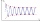The sum of four consecutive natural numbers is 114. Find them.
• The sumThe sum of the first 10 members of the arithmetic sequence is 120. What will be the sum if the difference is reduced by 2?
• Two geometric progressions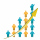Insert several numbers between numbers 6 and 384 so that they form with the given GP numbers and that the following applies: a) the sum of all numbers is 510 And for another GP to apply: b) the sum of entered numbers is -132 (These are two different geome
• Prime divisorsFind 2/3 of the sum's ratio and the product of all prime divisors of the number 120.
• Reminder and quotientThere are given the number C = 281, D = 201. Find the highest natural number S so that the C:S, D:S are with the remainder of 1,